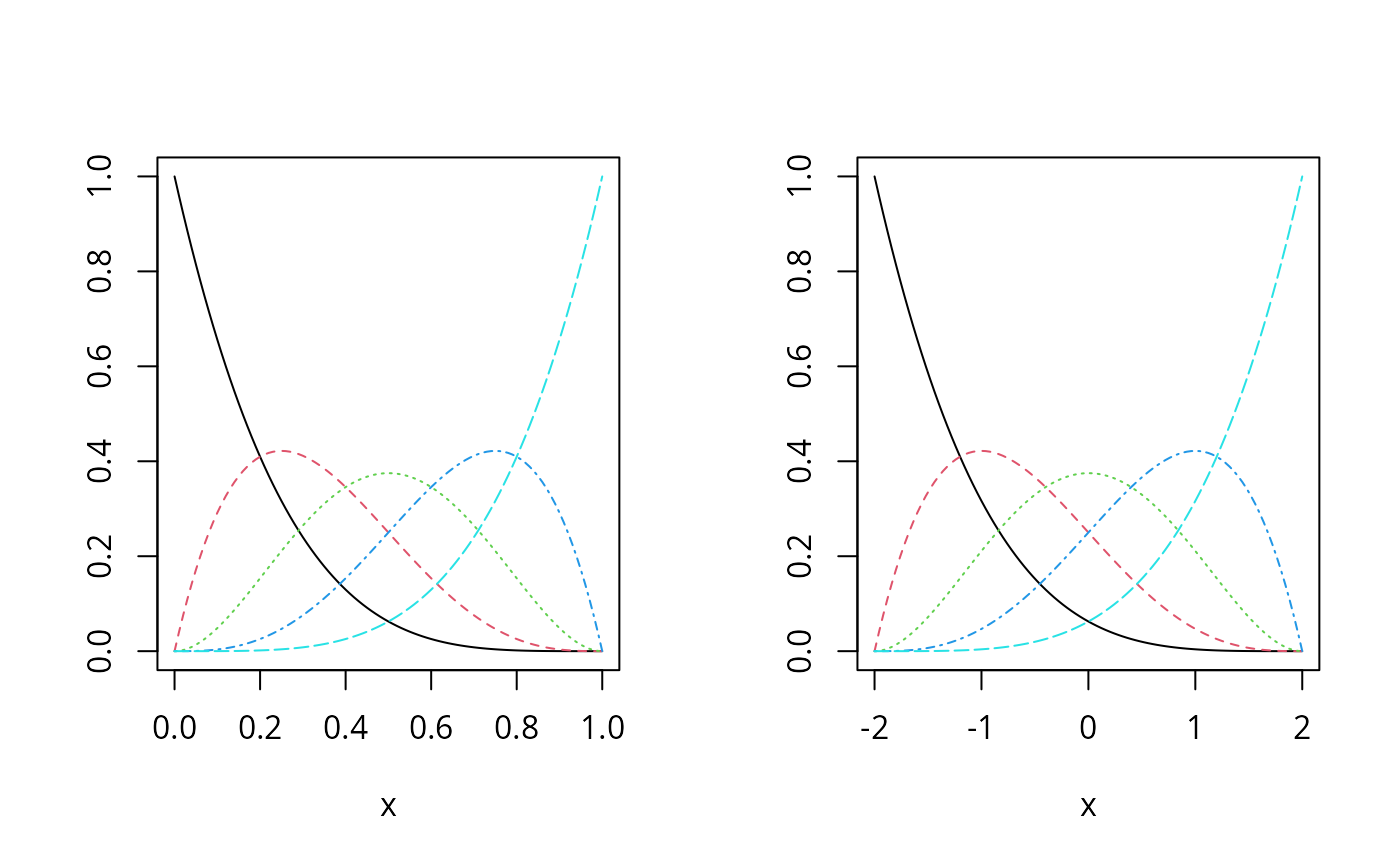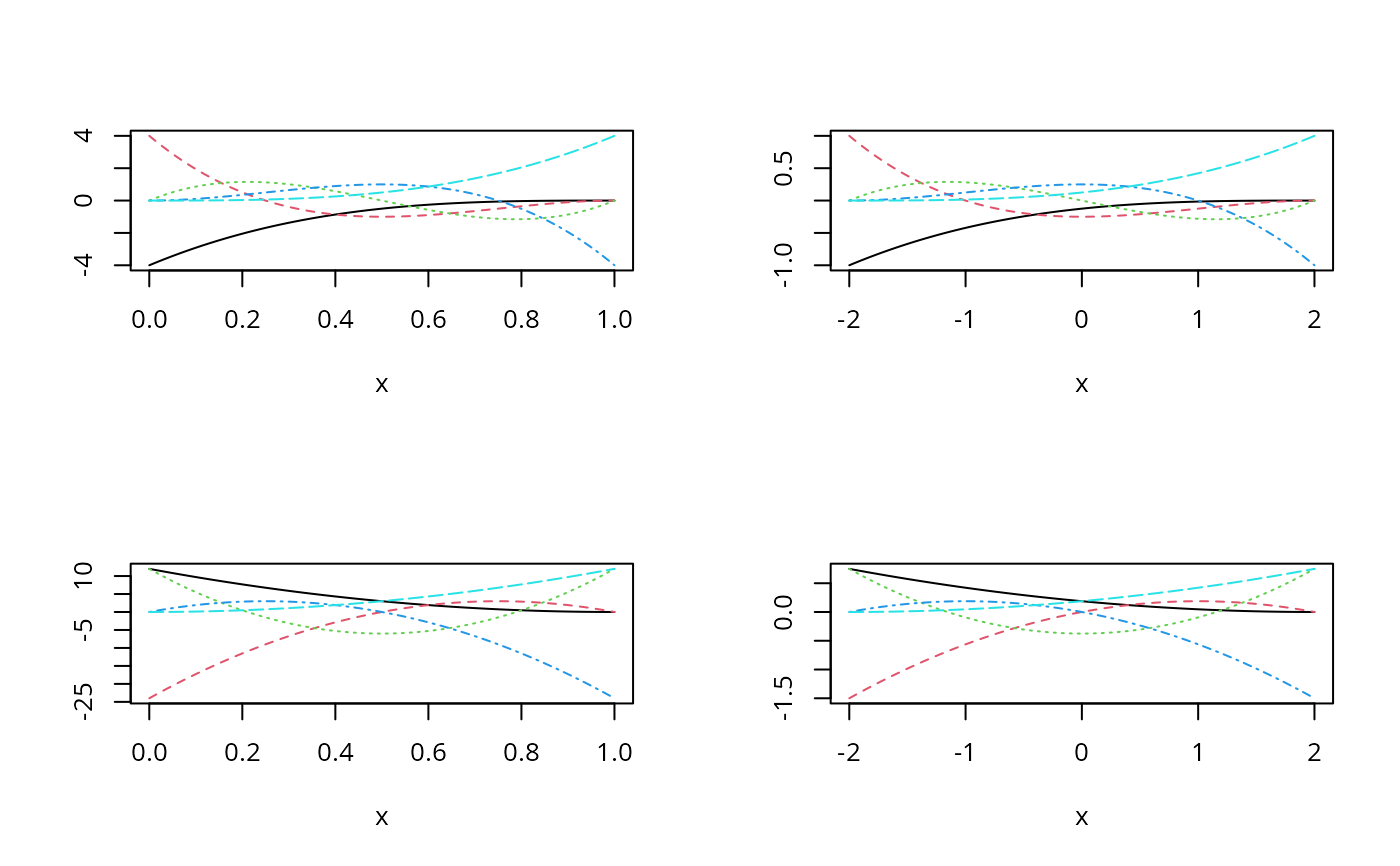Returns generalized Bernstein polynomial basis functions of the given degree over the specified range.

## Usage

bernsteinPoly(
x,
degree = 3,
intercept = FALSE,
Boundary.knots = NULL,
derivs = 0L,
integral = FALSE,
...
)

bpoly(
x,
degree = 3,
intercept = FALSE,
Boundary.knots = NULL,
derivs = 0L,
integral = FALSE,
...
)

## Arguments

x

The predictor variable taking values inside of the specified boundary. Missing values are allowed and will be returned as they are.

degree

A nonnegative integer representing the degree of the polynomials.

intercept

If TRUE, the complete basis matrix will be returned. Otherwise, the first basis will be excluded from the output.

Boundary.knots

Boundary points at which to anchor the Bernstein polynomial basis. The default value is NULL and the boundary knots is set internally to be range(x, na.rm = TRUE).

derivs

A nonnegative integer specifying the order of derivatives. The default value is 0L for Bernstein polynomial basis functions.

integral

A logical value. If TRUE, the integrals of the Bernstein polynomials will be returned. The default value is FALSE.

...

Optional arguments that are not used.

## Value

A BernsteinPoly object that is essentially a numeric matrix of dimension length(x) by degree + as.integer(intercept).

## Details

The Bernstein polynomial basis functions are defined over the support from 0 to 1. The generalized Bernstein polynomial basis functions extend the support to any finite interval in the real line.

The function bpoly() is an alias to encourage the use in a model formula.

## Examples

library(splines2)

x1 <- seq.int(0, 1, 0.01)
x2 <- seq.int(- 2, 2, 0.01)

## Bernstein polynomial basis matrix over [0, 1]
bMat1 <- bernsteinPoly(x1, degree = 4, intercept = TRUE)

## generalized Bernstein polynomials basis over [- 2, 2]
bMat2 <- bernsteinPoly(x2, degree = 4, intercept = TRUE)

op <- par(mfrow = c(1, 2))
plot(bMat1)
plot(bMat2)## the first and second derivative matrix
d1Mat1 <- bernsteinPoly(x1, degree = 4, derivs = 1, intercept = TRUE)
d2Mat1 <- bernsteinPoly(x1, degree = 4, derivs = 2, intercept = TRUE)
d1Mat2 <- bernsteinPoly(x2, degree = 4, derivs = 1, intercept = TRUE)
d2Mat2 <- bernsteinPoly(x2, degree = 4, derivs = 2, intercept = TRUE)

par(mfrow = c(2, 2))
plot(d1Mat1)
plot(d1Mat2)
plot(d2Mat1)
plot(d2Mat2)## reset to previous plotting settings
par(op)

## or use the deriv method
all.equal(d1Mat1, deriv(bMat1))
#>  TRUE
all.equal(d2Mat1, deriv(bMat1, 2))
#>  TRUE

## the integrals
iMat1 <- bernsteinPoly(x1, degree = 4, integral = TRUE, intercept = TRUE)
iMat2 <- bernsteinPoly(x2, degree = 4, integral = TRUE, intercept = TRUE)
all.equal(deriv(iMat1), bMat1, check.attributes = FALSE)
#>  TRUE
all.equal(deriv(iMat2), bMat2, check.attributes = FALSE)
#>  TRUE